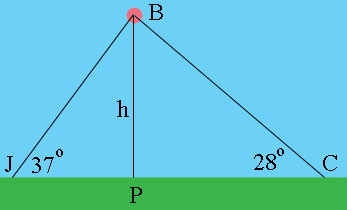SEARCH HOMEMath Central Quandaries & QueriesQuestion from C, a teacher: Chelcy and Jorgeare 2.32 miles apart. The observe a hot air balloon directly overhead between them Chelcy's angle of elevation is 28 degrees. Jorge's Angle of elevation is 37 degrees. What is the altitude of the balloon?Hi,

I drew a diagram. (Not to scale.)Chelcy is at C, Jorgeare is at J, P is the point between them directly below the balloon B and h is the height of the balloon in miles. Suppose the distance from J to P is x miles. What is he distance from P to C?

$\tan( 37^o) = \frac{h}{x}.$ What is $\tan(28^o)?$ Solve the first equation for $x$ and substitute into the second equation. Solve for $h.$

PennyMath Central is supported by the University of Regina and The Pacific Institute for the Mathematical Sciences.